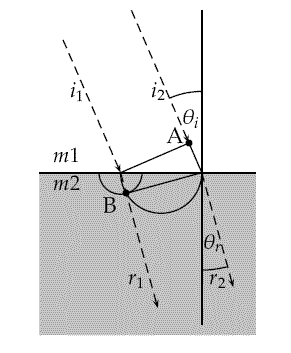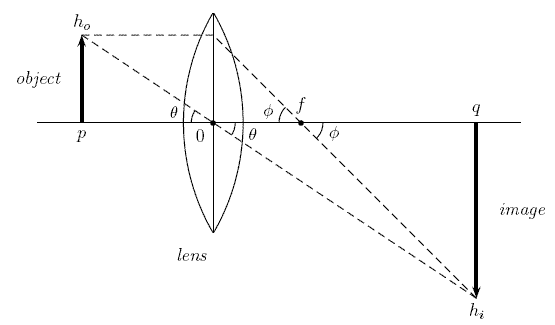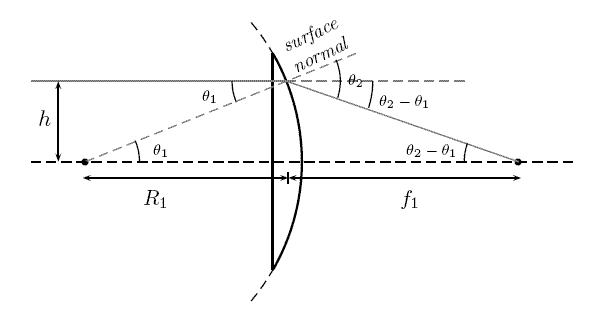﻿ Optics

# Optics and Lenses

This page contains references to various papers dealing with optics. In particular, lenses, microscopes, telescopes and other optical instruments are considered.

## Snell's Law

There are a number of ways to prove Snell's law. A purely geometric proof is provided here.Refraction Diagram for Snell's Law

## Finding the Focal Point of a Spherical Mirror or Lens

A paper deriving the relationship between the radius of curvature and the focal point of spherical optical surface is presented here:  focalpt.pdf

## Derivation of Lens RelationsLens, Object and Image

In elementary discussions of lenses it is stated that the following equation holds:

1/f = 1/p + 1/q

A proof is given in lensfoc.pdf .

## Lensmaker's Formula

The lensmaker's formula related the focal point of a lens with the radii of curvature and the index of refraction. A paper deriving the formula is here lensmaker.pdfRay Diagram for Analysis